# 15-344/Homework Assignment 7

Jump to: navigation, search

This assignment is due at the tutorials on Thursday November 19. Here and everywhere, neatness counts!! You may be brilliant and you may mean just the right things, but if the teaching assistants will be having hard time deciphering your work they will give up and assume it is wrong.

Reread sections 5.1-5.4 of our textbook. Remember that reading math isn't like reading a novel! If you read a novel and miss a few details most likely you'll still understand the novel. But if you miss a few details in a math text, often you'll miss everything that follows. So reading math takes reading and rereading and rerereading and a lot of thought about what you've read. Also, preread sections 5.5 and 6.1, just to get a feel for the future.

Solve problems 8, 10, 15, 20, 24, 28, and 29 in section 5.3 and problems 1, 3, 16, 39, 40, 63, and 65 in section 5.4, but submit only your solutions of the underlined problems.

 Dror's notes above / Students' notes below

Homework Assignment 7 Solution

15 How many 8-digit sequences are there involving exactly six different digits?

A) We have 2 cases: 1 digit repeated three times (for example, 11123456) and 2 digits repeated (for example, 11223456)

For the first case, there are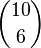$\binom{10}{6}$ ways to choose 6 digits from 10 digit choice. Then there are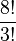$\frac{8!}{3!}$ arrangements involving a triple digit and and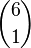$\binom{6}{1}$ different triple digits possible (111,222 and so on).So, in total, we have: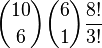$\binom{10}{6} \binom{6}{1}\frac{8!}{3!}$

Similarly, for the second case, we have: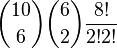$\binom{10}{6} \binom{6}{2}\frac{8!}{2!2!}$

The sum of the two cases gives the answer: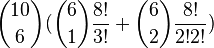$\binom{10}{6}(\binom{6}{1}\frac{8!}{3!}+ \binom{6}{2}\frac{8!}{2!2!})$

24 How many arrangements of the word PREPOSTEROUS are there in which the five vowels are consecutive?

A)

We have P-2, R-2, E-2, O-2, S-2, T-1,U-1

The vowels are OOUEE and its variations.

The number of all the possible variations of OOUEE is: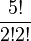$\frac{5!}{2!2!}$

The remaining 7 letters can be arranged in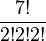$\frac{7!}{2!2!2!}$ ways.

Also, there are 8 different positions for the vowel group in the word. For example, OOUEE_ _ _ _ _ _ _ and _ _ _ _ _ _ _ OOUEE

In total,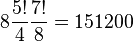$8\frac{5!}{4}\frac{7!}{8} = 151200$

28 How many ways are there to place nine different rings on the four fingers of your right hand if a) the order of the rings on a finger does not matter b) the order of the rings on a finger is considered

A) a) For each ring, we have a choice of being on 1 - 4th finger. So, we have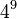$4^9$ possible such ways.

A) b) First, without considering the different types of the rings, consider the distribution of the nine rings to four fingers: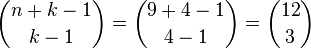$\binom{n+k-1}{k-1} = \binom{9+4-1}{4-1} = \binom{12}{3}$ If we consider the fact that the rings are all different, we have the permutations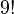$9!$ So, in total,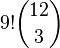$9!\binom{12}{3}$ ways.